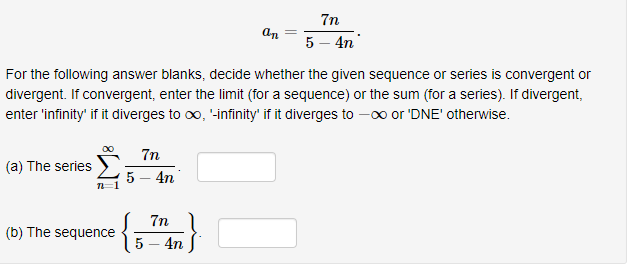# 7n5-4nFor the following answer blanks, decide whether the given sequence or series is convergent ordivergent. If convergent, enter the limit (for a sequence) or the sum (for a series). If divergent,enter 'infinity' if it diverges to co, -infinity' if it diverges to -oo or 'DNE' otherwise.(a) The serie 5 4T(b) The sequence5-4n

Question
45 viewshelp_outlineImage Transcriptionclose7n 5-4n For the following answer blanks, decide whether the given sequence or series is convergent or divergent. If convergent, enter the limit (for a sequence) or the sum (for a series). If divergent, enter 'infinity' if it diverges to co, -infinity' if it diverges to -oo or 'DNE' otherwise. (a) The serie 5 4T (b) The sequence 5-4n fullscreen
check_circle

Step 1

(a)

we are given a series as

Step 2

We can use divergence test

So, we can find limit of the nth term

Step 3

Since, the value of limit is finite

So, by diver...

### Want to see the full answer?

See Solution

#### Want to see this answer and more?

Solutions are written by subject experts who are available 24/7. Questions are typically answered within 1 hour.*

See Solution
*Response times may vary by subject and question.
Tagged in

### Calculus